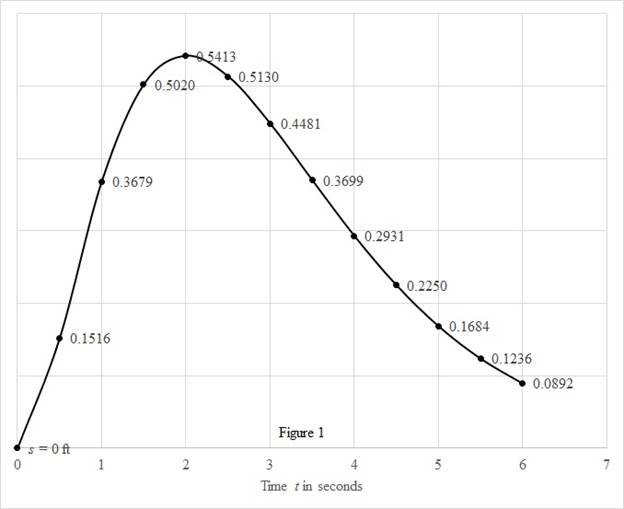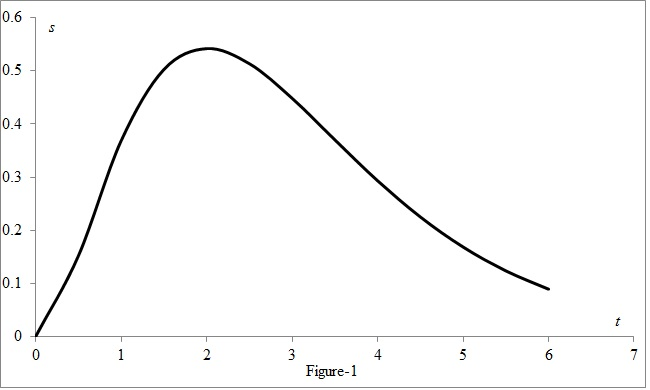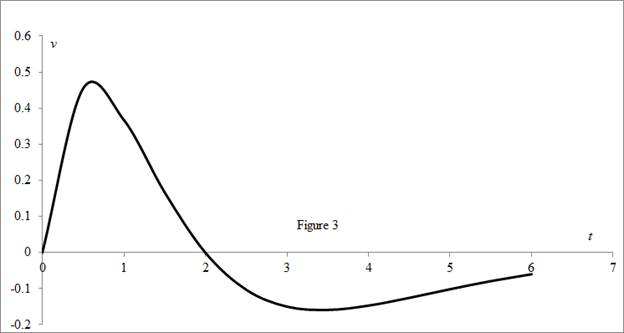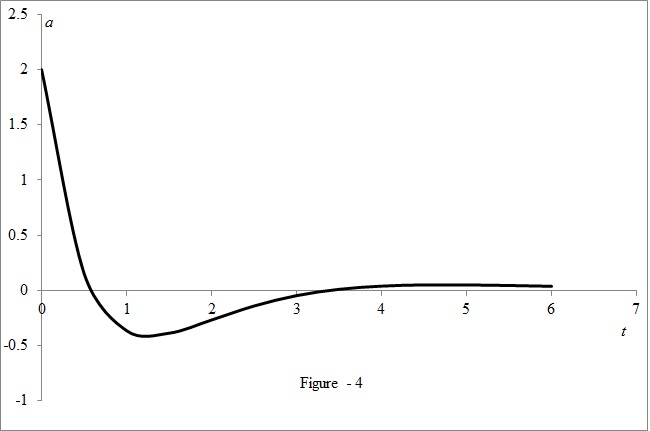# The velocity at time t .### Single Variable Calculus: Concepts...

4th Edition
James Stewart
Publisher: Cengage Learning
ISBN: 9781337687805### Single Variable Calculus: Concepts...

4th Edition
James Stewart
Publisher: Cengage Learning
ISBN: 9781337687805

#### Solutions

Chapter 3.8, Problem 4E

(a)

To determine

## To find: The velocity at time t.

Expert Solution

The velocity at time is f'(t)=tet(2t)ft/sec_.

### Explanation of Solution

Given:

The given equation is as below.

s=f(t)=t2et (1)

Calculation:

Calculate the velocity at time t.

Differentiate the equation (1) with respect to time.

v(t)=f'(t)=t2(et)+et(2t)=ett2+2tet

f'(t)=tet(2t) (2)

Therefore, the velocity at time t is f'(t)=tet(2t)ft/sec_.

(b)

To determine

### To find: The velocity after 1 second.

Expert Solution

The velocity after 1 second is v(1)=0.3678ft/sec_.

### Explanation of Solution

Calculate the velocity after 1 second.

Substitute 1 for t in the equation (2).

f'(t)=tet(2t)f'(1)=1e1(21)=0.3678ft/s

Therefore, the velocity after 1 second is v(1)=0.3678ft/sec_.

(c)

To determine

### To find: The time when particle at rest.

Expert Solution

The particle never is at rest when 0<t<2sec_

### Explanation of Solution

Calculate the time when particle will be at rest.

The velocity will be zero, when the particle is at rest.

Substitute 0 for v(t) in the equation (2).

v(t)=tet(2t)0=tet(2t)t=0sec2t=0t=2sec

Therefore, the particle at rest when t=0sec_ and t=2sec_.

(d)

To determine

### To find: The particle moving in the positive direction.

Expert Solution

The particle will be moving in the positive direction within the time limits 0<t<2sec and t>2sec.

### Explanation of Solution

Calculate the time during which the particle will be moving in the positive direction.

If the particle moves in positive direction, the velocity at any time t will be greater than zero.

v(t)=tet(2t)>00<t<2;t>2

Therefore, the particle will be moving in the positive direction within the time limits 0<t<2sec and t>2sec.

(e)

To determine

### To find: The total distance traveled during the first 6 seconds.

Expert Solution

The total distance travelled during first 6 second is f(6)=0.0892ft_.

### Explanation of Solution

Calculate the total distance traveled during first 6 seconds.

Substitute 6 for t in the equation (1).

f(t)=t2etf(6)=(62)e6f(6)=0.0892ft

Therefore, the total distance travelled during first 6 seconds is f(6)=0.0892ft_.

(f)

To determine

### To find: The diagram to illustrate the motion of the particle.

Expert Solution

The diagram is shown in the figure (1).

### Explanation of Solution

Show the diagram to illustrate the motion of the particle as shown below in figure (1).(g)

To determine

### To find: The acceleration at time t and after 1 second.

Expert Solution

The acceleration at time is et(24t+t2)ft/s2_ and after one second is 0.368ft/s2_.

### Explanation of Solution

Calculate the acceleration at time t.

Differentiate the equation (2) with respect to t.

f"(t)=2et4ett+ett2f"(t)=et(24t+t2)

Therefore, the acceleration at time is et(24t+t2)ft/s2_.

Calculate the acceleration after 1 second.

Substitute 1 for t in the above equation.

f"(t)=et(24t+t2)f"(1)=e(1)(24(1)+(1)2)=0.368(1)

f"(1)=0.368ft/s2

Therefore, the acceleration after 1 second is 0.368ft/s2_.

(h)

To determine

### To sketch: The graph the position, velocity, and acceleration function for 0≤t≤6.

Expert Solution

The position, velocity, and acceleration functions are plotted for time limits 0t6 in figures (2), (3), and (4).

### Explanation of Solution

Calculate the position using the formula.

s=f(t)=t2et

Substitute 0 for t in the above equation.

f(t)=t2etf(0)=(0)2e0=0

Similarly, calculate the remaining values.

Tabulate the value of t and f(t) as shown in the table (1).

 t s=f(t)=t2e−t 0 0 0.5 0.15163 1 0.36788 1.5 0.50204 2 0.54134 2.5 0.51303 3 0.44808 3.5 0.36992 4 0.29305 4.5 0.22496 5 0.16845 5.5 0.12362 6 0.08924

Calculate the velocity using the expression.

f'(t)=tet(2t)

Substitute 0 for t in the above equation.

f'(t)=tet(2t)f'(0)=(0)e0(20)=0

Similarly, calculate the remaining values.

Tabulate the value of t and v(t) as shown in the table (2).

 t f'(t)=te−t(2−t) 0 0 0.5 0.4549 1 0.36788 1.5 0.16735 2 0 2.5 -0.1026 3 -0.1494 3.5 -0.1585 4 -0.1465 4.5 -0.125 5 -0.1011 5.5 -0.0787 6 -0.0595

Calculate the acceleration using the formula.

f"(t)=et(24t+t2)

Substitute 0 for t in the above equation.

f"(t)=et(24t+t2)f"(0)=e0(24(0)+(0)2)=2

Similarly, calculate the remaining values.

Tabulate the value of t and a(t) as shown in the table (3).

 t f"(t)=e−t(2−4t+t2) 0 2 0.5 0.15163 1 -0.3679 1.5 -0.3905 2 -0.2707 2.5 -0.1436 3 -0.0498 3.5 0.00755 4 0.03663 4.5 0.04721 5 0.04717 5.5 0.04189 6 0.0347

Draw the position as a function of time curve as shown in the figure (2).Draw the speed as a function of time curve as shown in the Figure (3).Draw the acceleration as a function of time curve as shown in the Figure (4).(i)

To determine

### To find: The time when the particle is speeding up and slowing down.

Expert Solution

The acceleration is positive when the value of time t is greater than 1.30sec and till 3.41 seconds. This will be increasing in speed. The acceleration is negative when the value of time is lesser than 1.3sec and this will be slowing down. 3.41 seconds. The acceleration is positive when the value of time t is greater than 3.41sec and till 4.5 seconds. This will be increasing in speed.

### Explanation of Solution

Calculate the time when particle is speeding up and slowing down.

Substitute 0 for f"(t) in the equation f"(t)=et(24t+t2).

0=et(24t+t2)t=3.41sec

Substitute 1sec for t in the equation f"(t)=et(24t+t2).

f"(1)=e1(24×1+12)=0.368<0

Substitute 4sec for t in the equation f"(t)=et(24t+t2).

f"(4)=e4(24×4+42)=0.0366>0

Therefore, the acceleration is positive when the value of time t is greater than 1.30sec and till 3.41 seconds. This will be increasing in speed. The acceleration is negative when the value of time is lesser than 1.3sec and this will be slowing down. 3.41 seconds. The acceleration is positive when the value of time t is greater than 3.41sec and till 4.5 seconds. This will be increasing in speed.

### Have a homework question?

Subscribe to bartleby learn! Ask subject matter experts 30 homework questions each month. Plus, you’ll have access to millions of step-by-step textbook answers!